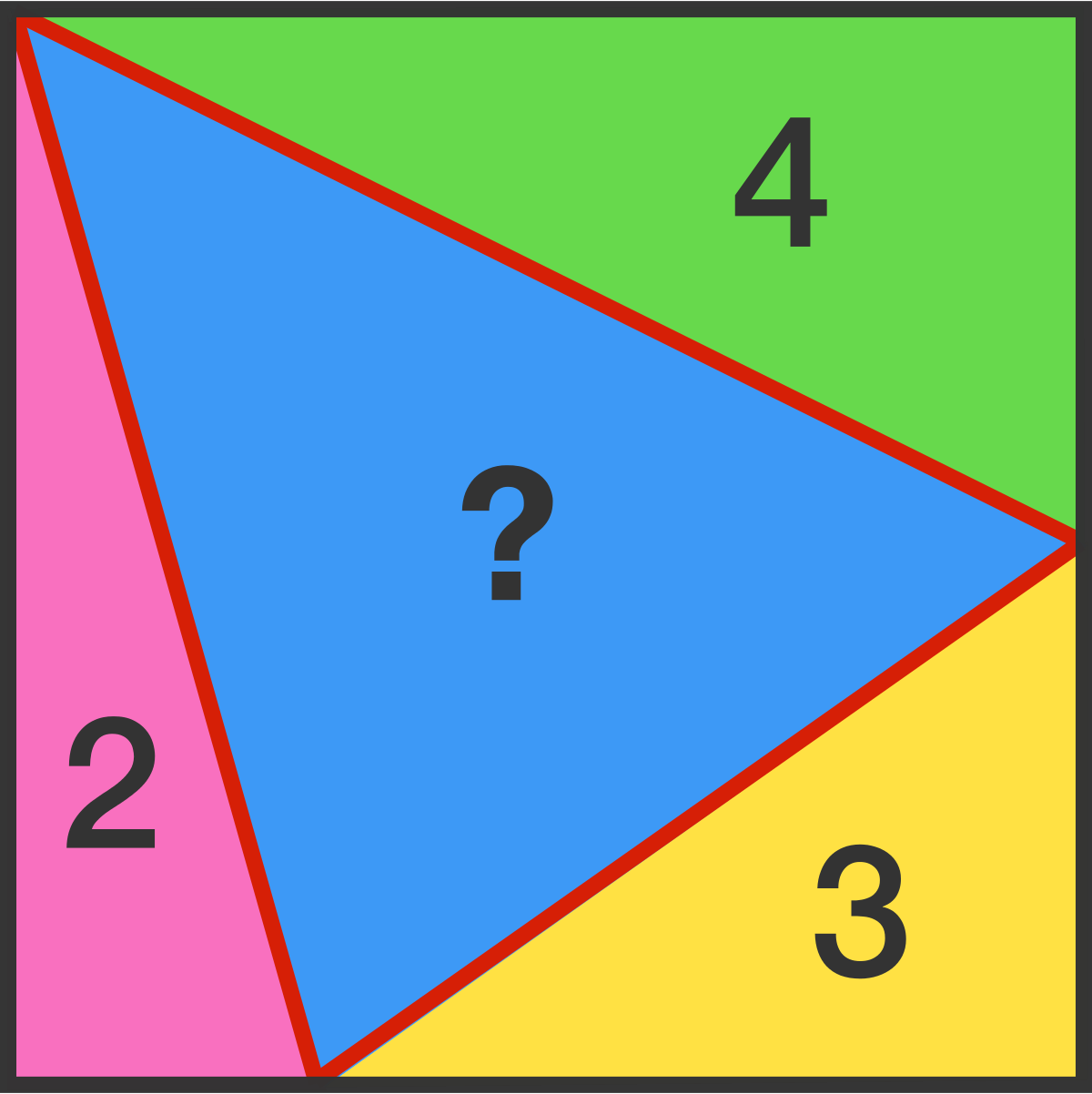Cody

Problem 44267. Brain Teaser Solver

Write an algorithm to solve this brain teaser:

You have a square that is broken into four triangles, the area of three of those triangles is given. You must determine the area of the center triangle. Your function will take in 3 arguments A, B, and C. Below is an image of this problem, in this example A = 4, B = 3, and C = 2.Solution Stats

45.16% Correct | 54.84% Incorrect
Last solution submitted on Apr 27, 2019